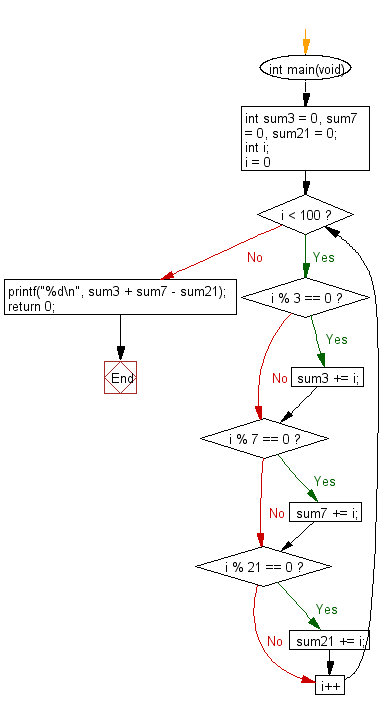﻿ C Program: Sum of all the multiples of 3 or 7 below 100# C Exercises: Find the sum of all the multiples of 3 or 7 below 100

## C Programming Practice: Exercise-17 with Solution

The natural numbers below 10 that are multiples of 3 or 7 are 3, 7, 6 and 9. The sum of these multiples is 25.
Write a C programming to find the sum of all the multiples of 3 or 7 below 100.

C Code:

``````#include <stdio.h>
int main(void)
{
int sum3 = 0, sum7 = 0, sum21 = 0;
int i;
for (i = 0; i < 100; i++) {
if (i % 3 == 0) {
sum3 += i;
}
if (i % 7 == 0) {
sum7 += i;
}
if (i % 21 == 0) {
sum21 += i;
}
}
printf("%d\n", sum3 + sum7 - sum21);
return 0;
}
``````

Sample Output:

```2208
```

Flowchart:## C Programming Code Editor:

What is the difficulty level of this exercise?

Test your Programming skills with w3resource's quiz.

﻿

## C Programming: Tips of the Day

What is the difference between printf() and puts() in C?

puts is simpler than printf but be aware that the former automatically appends a newline. If that's not what you want, you can fputs your string to stdout or use printf.

Ref :https://bit.ly/3f2fwcH